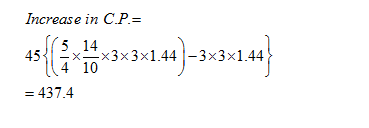• This is an assessment test.
• These tests focus on geometry and mensuration and are meant to indicate your preparation level for the subject.
• Kindly take the tests in this series with a pre-defined schedule.

## Geometry and Mensuration: Test 4

Congratulations - you have completed Geometry and Mensuration: Test 4. You scored %%SCORE%% out of %%TOTAL%%. You correct answer percentage: %%PERCENTAGE%% . Your performance has been rated as %%RATING%%
 Question 1
What is the area of a circle whose circumference is 1047.2 metres?
 A 87301.67 sq. meters B 85142.28 sq. metres C 79943.82 sq. metres D 78621.47 sq. metres
Question 1 Explanation:
$\begin{array}{l}2\pi r=1047.2\\r=1047.2\times \frac{7}{2\times 22}=\frac{500}{3}\\\pi {{r}^{2}}=\frac{\frac{22}{7}\times \frac{500}{3}\times 500}{3}=87301\end{array}$
 Question 2
An order was placed for supply of carpet of breadth 3 metres, the length of carpet was 1.44 times of breadth. Subsequently the breadth and length were increased by 25 and 40 per cent respectively. At the rate of Rs.45 per square metre, what would be the Increase In the cost of the carpet?
 A Rs.1020.6 B Rs.398.8 C Rs.437.4 D Rs.583.2
Question 2 Explanation:
The original breadth and length of the carpet is 3m and 3 × 1.44 m respectively.Question 3
The length of a rectangular plot is thrice its breadth. If the area of the rectangular plot is 7803 sq. metre, what is the breadth of the rectangular plot? Let the breadth is 'b' and the length is '3b'. Area = 3b × b = 7803 =>3b2 = 7803 =>b2 = 2601 => b = 51 m. Correct option is (a)
 A 51 metres B 153 metres C 104 metres D 88 metres
Question 3 Explanation:
Let the breadth is 'b' and the length is '3b'.
Area = 3b × b = 7803
=>3b2 = 7803
=>b2 = 2601 => b = 51 m. Correct option is (a)
 Question 4
The cost of fencing a circular plot at the rate of Rs.15 per metre is Rs.3, 300. What will be the cost of flooring the plot at the rate of Rs.100 per square metre?
 A Rs.3, 85, 000 B Rs.2, 20, 000 C Rs.3, 50, 000 D Cannot be determined
Question 4 Explanation:
$\displaystyle \begin{array}{l}Circumference\text{ }of\text{ }circle\text{ }=\text{ }3300/15\text{ }=\text{ }220m\\\frac{44}{7}r=220\\r=35\\Area=\pi {{r}^{2}}\\\frac{22}{7}\times 35\times 35=3850\end{array}$
Hence the cost of flooring = 3850 × 100 = Rs 385000.
 Question 5
If the area of a circle is 616 cm2, what is its Circumference?
 A 76 cm. B 84 cm. C 96 cm. D none
Question 5 Explanation:
Let 'r' be the radius of the circle.
Area of circle
$\displaystyle \begin{array}{l}\pi {{r}^{2}}=616\\r=\sqrt{196}=14\\2\pi r=88m\end{array}$
Once you are finished, click the button below. Any items you have not completed will be marked incorrect.
There are 5 questions to complete.
 ← List →